New update is available. Click here to update.
Last Updated: Apr 1, 2023
Medium

# Check if an Array can be Divided into Pairs Whose Sum is Divisible by KAuthor Ayushi Goyal0 upvotes

## Introduction

In this article, we will discuss a problem to check if an array can be divided into pairs whose sum is divisible by k. We will briefly discuss the algorithm used, its implementation, and time and space complexity. Before discussing how to check if an array can be divided into pairs whose sum is divisible by k, let’s first discuss what an array means.

Array: It is a linear data structure consisting of a collection of values called elements of an array, stored in a contiguous memory location, and can be accessed using array indexes.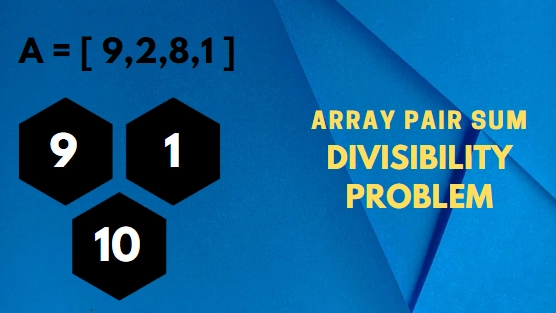In this problem, we are given an array of any size(provided by the user) and a number ‘k’, and our task is to write a function that will check if an array can be divided into pairs whose sum is divisible by k, and return true or false accordingly.

Let's have an example to make the problem statement clear.

Example:

In this example, we have an array, ‘arr’, and the value of the number ‘k’ = 5. The image below shows all the pairs whose sum is multiple of ‘k’.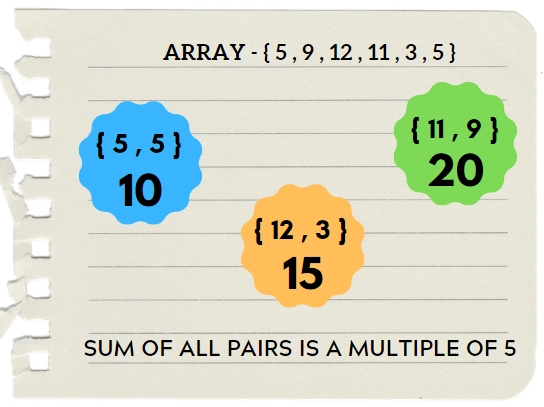## Algorithm🕵️‍♀️

• Take array size as input ‘n’.

• Create an array of size ‘n’, say, ‘arr[n]’.

• Take a variable ‘k’ input from the user.

• Check if array size is odd (‘n’%2 != 0), return false.

• Take an unordered map ‘m’.

• Run a for loop from ‘i’ = 0 to ‘n’ and store the occurrence of all reminders in the map obtained by ‘((arr[i] % k) + k) % k ’

• Run another for loop from ‘i’ = 0 to ‘n’. Inside this loop, do the following operations:-

i. Take a temporary variable ‘reminder’ and store  reminder of current element using ‘((arr[i] % k) + k) % k’.

ii. Check if ‘reminder’ is double of ‘k’.

iii. If true, check if this ‘reminder’ frequency is odd, then return false.

iv. Otherwise, check if ‘reminder’ is zero and the frequency of ‘reminder’ is odd, then return false.

v. Otherwise, check if the frequency of ‘reminder’ is not equal to the frequency of ‘k’ - ‘reminder’, then return false.

• If all the above conditions are not satisfied, then return true.

For example, the input array is Arr[]={12, 3, 5, 3, 6, 1} and the value of K = 6

So, firstly we will check if the array size is odd then numbers cannot be divided into pairs so just return false. Otherwise, we will store reminders using the formula ((arr[i] % k) + k) % k ’ for each element in the hash table. So our hash table will look like this: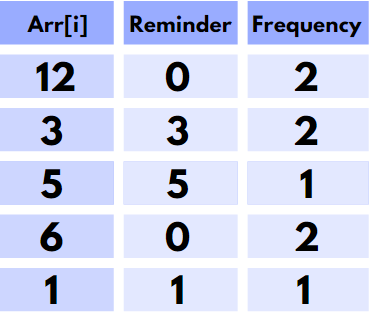As we will run a loop from ‘i’ = 0 to ‘n’, and in each pass, we have to go through the following set of conditions mentioned in the algorithm: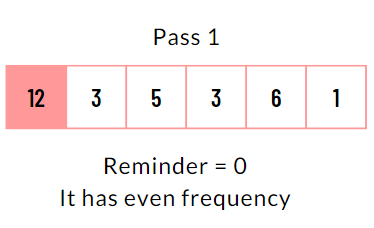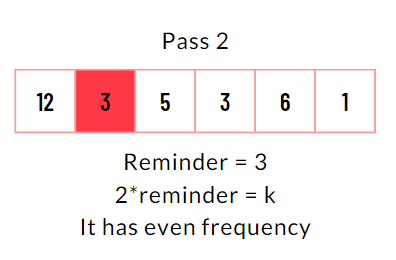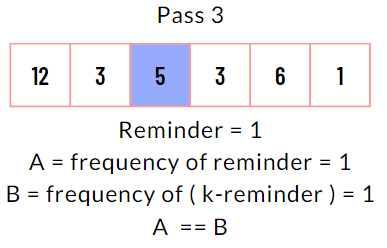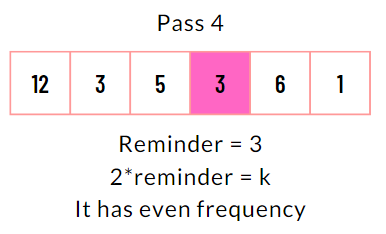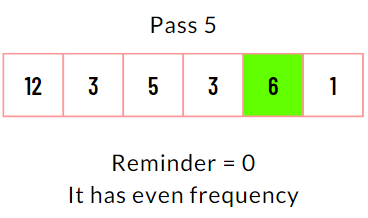So the final output is: Return ‘true’ as all conditions are false now and the loop ends.

## Implementation in C++💻👇

``````/*
C++ program → To check if an array can be divided into pairs whose sum is divisible by k
*/

#include <bits/stdc++.h>
using namespace std;

bool divisiblePairs(int arr[], int n, int k)
{
// Check is size of array is odd return false
if (n%2 != 0)
return false;

unordered_map<int, int> m;

// Count frequency of all remainders
for (int i = 0; i < n; i++)
m[((arr[i] % k) + k) % k]++;

// Traverse array to check if array can be divided or not.
for (int i = 0; i < n; i++)
{
int reminder = ((arr[i] % k) + k) % k;

// Check if reminder is double of k
if (2 * reminder == k)
if (m[reminder] % 2 != 0)
return false;

// Check if reminder is zero
else if (reminder == 0)
if (m[reminder]%2 != 0)
return false;

else if (m[reminder] != m[k - reminder])
return false;
}
return true;
}

int main()
{
int n;
cout<<"Enter array size : ";
cin>>n;

int a[n];
cout<<"\nEnter array elements : ";
for(int i=0;i<n;i++)
cin>>a[i];

int k;
cout<<"Enter value of K : ";
cin>>k;

if(divisiblePairs(a, n, k))
cout<<"Yes, Array can be divided.";
else
cout<<"No, array cannot be divided.";

return 0;
}``````

## Implementation in Java💻👇

``````/*
Java program → To check if an array can be divided into pairs whose sum is divisible by k
*/
import java.util.*;

class Main
{
static boolean divisiblePairs(int arr[], int n, int k)
{
// Check is size of array is odd return false
if(n%2 != 0)
return false;

Map<Integer, Integer>m = new HashMap<Integer, Integer>();
for(int i=0;i<n;i++)
{
if(m.containsKey(((arr[i] % k) + k) % k))
m.put( (((arr[i] % k) + k) % k), m.get(((arr[i] % k) + k) % k) + 1);
else
m.put(((arr[i] % k) + k) % k,1);
}

// Traverse array to check if array can be divided or not.
for (int i = 0; i < n; i++)
{
int reminder = ((arr[i] % k) + k) % k;

// Check if reminder is double of k
if (2 * reminder == k)
if (m.get(reminder) % 2 != 0)
return false;

// Check if reminder is zero
else if (reminder == 0)
if (m.get(reminder)%2 != 0)
return false;

else if (m.get(reminder) != m.get(k - reminder))
return false;
}
return true;
}

public static void main(String[] args)
{
Scanner sc=new Scanner(System.in);
System.out.print("Enter size of array : ");
int n=sc.nextInt();
int a[] = new int[n];
System.out.println("Enter elements of array : ");
for(int i=0; i<n; i++)
a[i] = sc.nextInt();

System.out.print("Enter value of K : ");
int k=sc.nextInt();

if (divisiblePairs(a, n, k))
System.out.println("Yes, Array can be divided.");
else
System.out.println("No, array cannot be divided.");
}
}``````

## Implementation in Python3💻👇

``````# Python3 program → To check if an array can be divided into pairs whose sum is divisible by k

from collections import defaultdict
def divisiblePairs(arr, n, k):
# Check is size of array is odd return false
if(n%2 != 0):
return 0

m = defaultdict(lambda: 0)
Store frequency of reminders of all element
for i in range(0, n):
m[((arr[i] % k) + k) % k] += 1

# Traverse array to check if array can be divided or not.
for i in range(0, n):
reminder = ((arr[i] % k) + k) % k

# Check if reminder is double of k
if (2 * reminder == k):
if (m[reminder] % 2 != 0):
return 0

# Check if reminder is zero
elif (reminder == 0):
if (m[reminder]%2 != 0):
return 0

elif (m[reminder] != m[k - reminder]):
return 0

return 1

if __name__=='__main__':
n=int(input("Enter size of array : "))
a=list(map(int, input("Enter array elements : ").strip().split()))
k = int(input("Enter value of k : "))

if divisiblePairs(a, n, k) == True:
print("Yes, Array can be divided.")
else:
print("No, array cannot be divided.")``````

## Time and Space Complexity⏳

We have used two sets of loops in the program.

• One is a single for loop iterating from 0 to ‘N’ to store the remainder of each element of one array in the map, and this will take O(N) time complexity.

• Another loop is a single loop used to check if an array can be divided into pairs whose sum is divisible by k, which will take O(N) time complexity.

🎀 Total time complexity = O(N) + O(N) = O(N)

🎀 Space Complexity = O(N), for an array of size N.

### What is an array?

An array is a linear data structure and a collection of similar values stored at contiguous memory locations. It is like a staircase, where each value is placed at every step, and elements can be accessed by knowing the stair number (or index number).

### What is Hashing?

Hashing is a technique using which a given value can be transformed into another value. It makes searching more accessible and quicker.

### What is the defaultdict in Python?

Defaultdict is a container and a subclass of the dictionary class. It is almost the same as dictionaries in Python, except that it doesn’t throw a key error. The default value of a defaultdict is NONE.

### What is the complexity to check if an array can be divided into pairs whose sum is divisible by k?

The time complexity for this problem is O(N) and space complexity is also O(N).

### Explain hashmap in java?

Hashmap in java is a part of Java’s collection library and is present in java.util package. It is used to store the data in a key-value pair. Duplicate values are replaced by the corresponding key already present in the hashmap.

Syntax:

``HashMap<Key, Value> identifier = new HashMap<>();``

## Conclusion

In this article, we have seen the implementation of the code to check if an array can be divided into pairs whose sum is divisible by k. We understood the problem by taking an example. We discussed an elaborative algorithm and the code for the same in C++, Java, and Python, along with the program's time and space complexity and output on user input using hashing.

Recommended problems -Please refer to our guided paths on Codestudio to learn more about DSA, Competitive Programming, Java Programming, and Operating System, etc. Have a look at the interview experiences and interview bundle for placement preparations. And also, enroll in our courses and refer to the mock test and problems available.

Happy Coding, Ninja!

Codekaze-June23 India's Biggest Tech Hiring Challenge is LIVE!Go on top